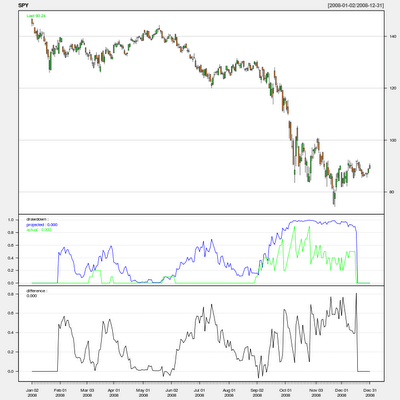Want to share your content on R-bloggers? click here if you have a blog, or here if you don't.

I’ve shown
several
examples
of how to use LSPM’s probDrawdown function as a
constraint when optimizing a leverage space portfolio.  Those posts implicitly assume the probDrawdown function produces an accurate estimate of actual drawdown.  This post will investigate the function’s accuracy.

Calculation Notes:
To calculate the probability of drawdown, the function traverses all permutations of the events in your lsp object over the given horizon and sums the probability of each permutation that hits the drawdown constraint.  The probability of each permutation is the product of the probability of
each event in the permutation.

In the example below, there are 20 events in each lsp object and the probability of drawdown is calculated over a horizon of 10 days, yielding 20^10 permutations to traverse – for each iteration.  So don’t be surprised when the code takes more than a couple minutes to run.

For a more detailed discussion about the calculation, see:
pp. 89-138 of The
, and/or
pp. 377-414 of The Handbook of Portfolio Mathematics
both by Ralph Vince.

Discussion:
The results below were run on daily SPY from 2008-01-01 to 2009-01-01, using 20 days of data to estimate the probability of a 5% drawdown over the next 10 days.  Results on daily QQQQ over the same period, and monthly SPX from 1950-present produced similar results.

I chose a prediction horizon of 10 periods to provide a fairly smooth actual probability of drawdown curve without making the probDrawdown calculation time too long.  Using 10 (instead of 20) days of data in the lsp object only changed results slightly.

The chart below shows that probDrawdown nearly always over estimates actual drawdown over the next 10 periods and hardly ever under estimates it.  While it’s comforting the function doesn’t under estimate risk, I would prefer a less biased estimator.Notice that the above calculation assumes each event is independently

distributed.  Brian Peterson
suggested a block
bootstrap
to attempt to preserve any dependence that may exist.  My
next post will investigate if that materially improves estimates.

Code:

library(quantmod)
library(LSPM)
library(snow)

# Pull data and calculate differences
symbol <- "SPY"
getSymbols(symbol, from=”2008-01-01″, to=”2009-01-01″)
sym <- get(symbol)
symDiff <- diff(Cl(sym))
symDiff <- 0 NP <- 20    # number of periods to use in lsp object
HR <- 10    # drawdown horizon
DD <- 0.05  # drawdown level # Initialize projected / actual drawdown objects
NR <- NROW(symDiff)
prjDD <- xts(rep(0,NR),index(symDiff))
actDD <- xts(rep(0,NR),index(symDiff)) # Socket cluster with snow to speed up probDrawdown()
cl <- makeSOCKcluster(2) # Start loop over data
for( i in (NP+1):(NR-HR) ) {

# Objects to hold data for the last 20 days and next 10 days
last20 <- symDiff[(i-NP):i]
next10 <- symDiff[(i+1):(i+HR)]
maxLoss <- -Cl(sym)[i]   # Portfolios to estimate drawdown and calculate actual drawdown
prjPort <- lsp(last20, f=1, maxLoss=maxLoss)
actPort <- lsp(next10, f=1, maxLoss=maxLoss)

# Estimate probability of drawdown
prjDD[i] <- probDrawdown(prjPort, DD, HR, snow=cl)

# Calculate actual drawdown probability
actDD[i] <- sum(HPR(actPort)/cummax(HPR(actPort)) <= (1-DD)) / HR
}
# End loop over data

# Chart results
drawdown=cbind(projected=prjDD,actual=actDD)
difference=prjDD-actDD
chartSeries(sym,log=FALSE,theme=’white’,name=symbol,